# How to Compute Difference Scores in SPSS

This quick tutorial will show you how to compute difference scores in SPSS, and save the results in a new variable.

Perhaps the most common scenario for computing difference scores is where you’ve got a pre-test/post-test scenario, and you want to see how the dependent variable has changed between the two conditions (difference scores are sometimes termed “change scores”).

### Quick Steps

1. Transform -> Compute Variable…
2. Name the variable to hold the new difference scores (in the Target Variable box)
3. Use the Numeric Expression box to calculate difference scores, using this format: Variable2Name – Variable1Name (or vice versa)
4. Click OK

## The Data

Our data for this tutorial comes from a hypothetical study looking at the effect of a new treatment for asthma by measuring the peak flow of a group of asthma patients before and after treatment.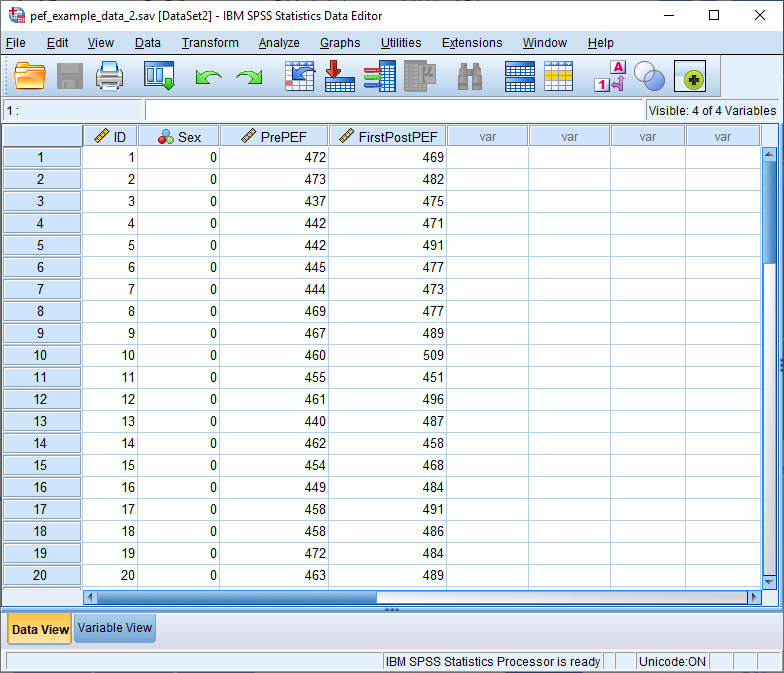The two variables we are interested in here are PrePEF – pretest peak expiratory flow (measured in litres per minute); and FirstPostPEF – posttest peak expiratory flow (measured in litres per minute).

We want to create an additional variable that holds the difference scores for these two variables allowing us to track how peak flow has changed after treatment.

## Compute Difference Scores

To begin, click Transform -> Compute Variable…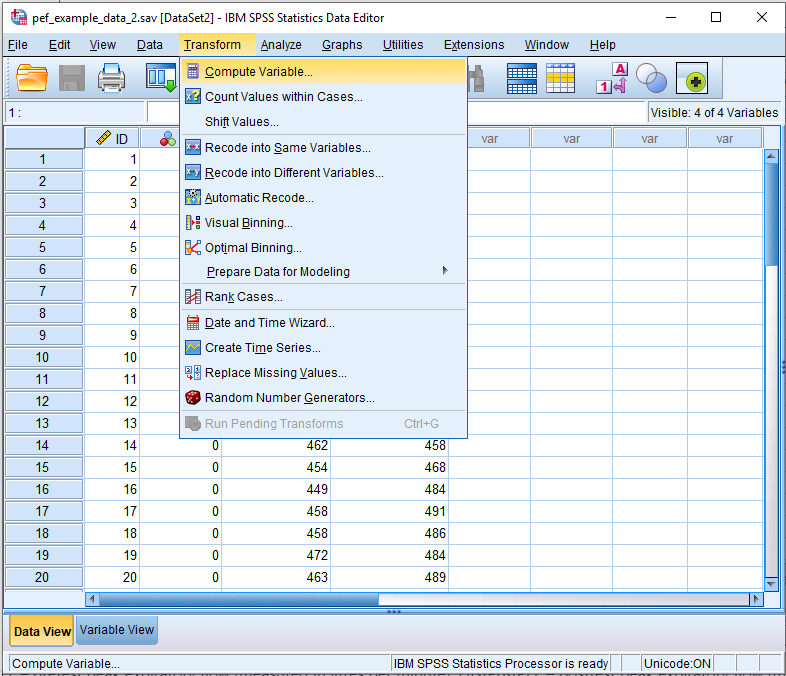This will bring up the Compute Variable dialog box.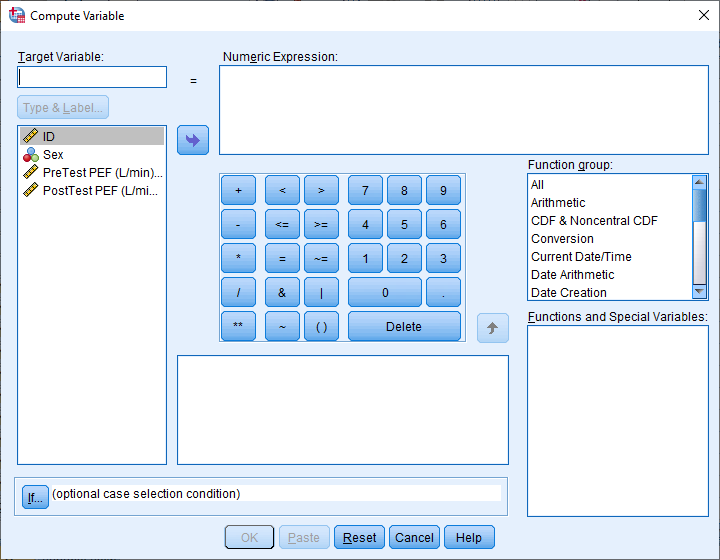This dialog enables us to create a new variable based on a variety of numeric (and other) operations. For example, suppose you have given your experimental subjects five different tests to complete, and you want to sum the scores of these tests for each subject, and fill a new variable with the totals. You can accomplish this task using the Compute Variable dialog box.

What we want to do here is to create a new variable that holds difference scores (or change scores) for our pretest and posttest variables. This is how the dialog box needs to be set up.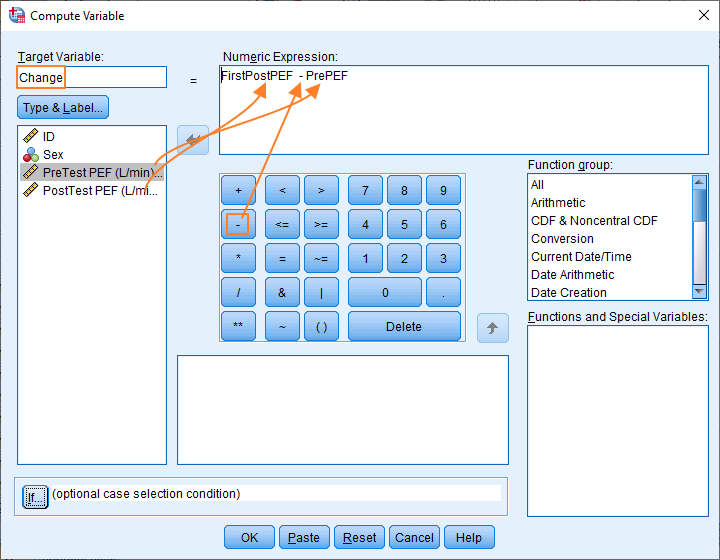This is fairly straightforward. To compute the difference scores we need to subtract the pretest score from the posttest score. It’s this way around because we want a positive number (representing an increase) if the posttest score is higher than the pretest score. We also need to name a new variable within which we’ll store our new difference scores.

As you can see above, we set up the calculation for the difference scores in the Numeric Expression box. Drag and drop the PostTestPEF variable into this box, then click the minus sign (on the keypad in the middle of the dialog box), and then drag and drop the PreTestPEF variable into the box.

Now you just need to type the name of the variable that’ll contain the difference scores in the Target Variable box. In our example, the new variable is called “Change”.

That’s all there is to it. Click the OK button to compute the difference scores and create a new variable.

## Result

The result of the procedure looks like this.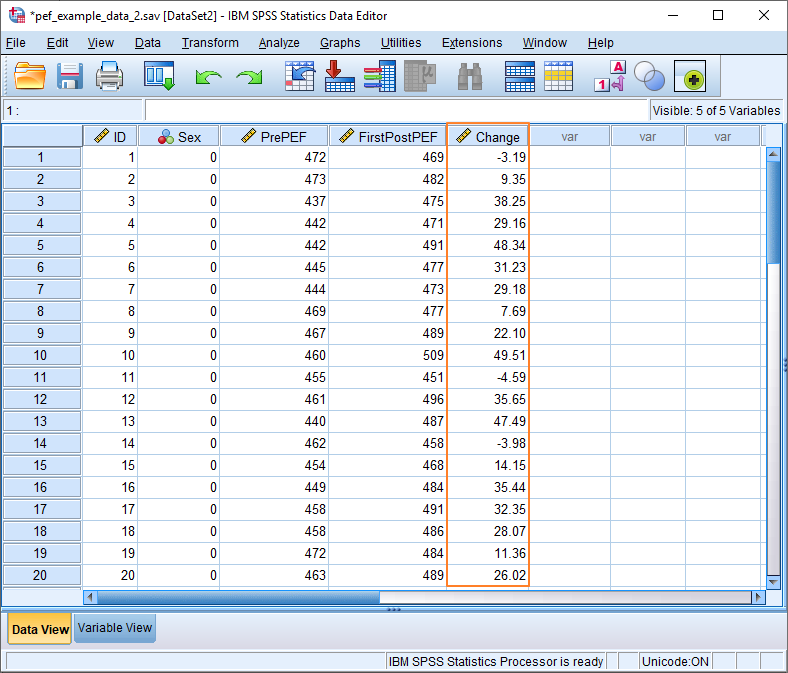As you can see, SPSS has created a new variable called “Change”, and filled it with difference scores (i.e., calculated by subtracting the PrePEF score from the FirstPostPEF score).

At this point it’s worth taking a look at the Variable View – just click on the tab towards the bottom of the screen – to check the properties of the variable that SPSS has created.

### Variable View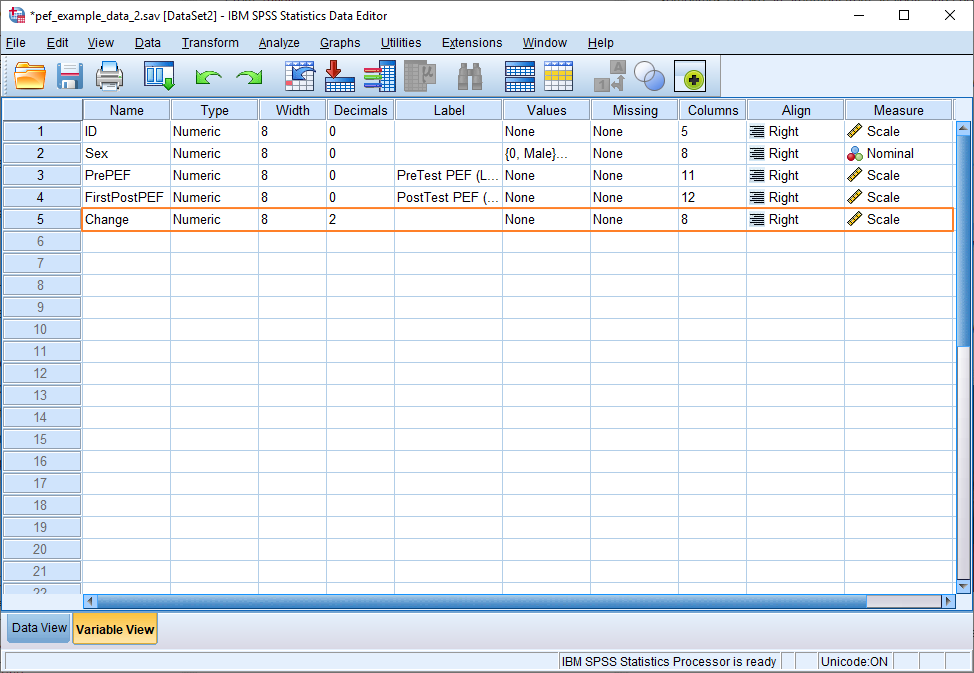This all looks okay. The type is Numeric and the level of measurement has been correctly identified as Scale.

The only thing we might want to alter is the number of decimals we’re going to display on the Data View. This is currently set at 2, whereas the other variables are configured to display without decimals. Click in the appropriate box if you want to change it. (We have a separate tutorial that deals with the Variable View in detail.)

***************

That’s pretty much it for this tutorial. You should now be able to use the Compute Variable option to calculate difference scores in SPSS. Obviously, this only begins to scratch the surface of the power of the numerical operations on offer via this menu item. We’ll look at some other common usages in future tutorials.